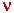Radar Guns and Einstein's Theories

My paper on the above subject: http://vixra.org/pdf/1806.0027v4.pdf
All my papers are at this link: http://vixra.org/author/edward_g_lake

This YouTube.com link has an interesting demonstration of a police radar:
https://www.youtube.com/watch?v=XlnYO_G_IxA

A paper at this link analyzes that video: http://www.ed-lake.com/radar-gun-video.pdf

The main question the video does not answer is:
How does the radar gun know that the ground isn't a target?

When the gun is stationary and is pointed at a moving target, it displays the speed of
the target (67 mph) in the "Target Speed" display on the left side:When the gun is moving and is pointed straight ahead, it displays the speed of
the radar gun (31 mph) in the "Patrol Speed" display on the right side:How does the gun know that it is moving?  How does the gun know that it is pointed at the ground and
not at a moving target just as it was in the first image?

My research indicates that the gun is NOT measuring the speed of the ground.  It is measuring its OWN speed.
It does that by bouncing photons off of the radome that covers the front of the gun.  The radome evidently
contains a few metallic particles that reflect a very small portion of the total number of photons emitted by the gun.The gun can then determine which photons bounced back from the radome and which bounced back
from the target.   It can do so by the fact that the radome photons return first, or by the fact that
the radome photons are always of the same quantity.

The key point is:  If the gun can measure its own speed by bouncing photons off of its own radome,
then a gun without a radome (or with a fully transparent radome) can measure its own speed when
inside a moving box truck by bouncing photons off the front or rear walls of the truck.
That clarifies the exact meaning of Einstein's Second Postulate:
"Light is always propagated in empty space with a definite velocity c
which is
independent of the state of motion of the emitting body."

See my paper on Radar Guns and Einstein's Theories for further details.

https://www.grc.nasa.gov/WWW/k-12/Numbers/Math/Mathematical_Thinking/how_do_police_radars.htm
The above page from NASA describes how a SINGLE PHOTON from a radar gun will get a reading on a speeding car.

Here is a copy of that page:

## How Do Police Radars Really Work?

Problem:
A car, traveling at speed, V, approaches a police radar, scanning for speeders with a frequency,. Calculate the approximate frequency shift of the reflected signal.

Solution:
Consider a single photon from the police radar. The photon must interact with the approaching car for a finite time while it is being reflected. Call this time,t. Let an interaction force, ± f, exist between the photon and the car for the time,t. The force exerted by the photon on the car, +f, acts to remove energy from the car. The force exerted by the car on the photon, -f, acts to add energy to the photon. Therefore, we expect the photon frequency to increase. During the timet, the car travels a distances = Vt. We may now write two equations, one for a change in momentum,p, and one for a change in energy,E:

 Momentum:p = ft 1
 Energy:E = fs = fVt 2

Since the photon energy, E, is equal to hn, where h = Planck's constant = 6.63 X 10-34 j sec, thenE = h3

Also, since photon momentum equals h/c, where c is the speed of light, thenp = 2h/c + h/c 4

where the first term on the RHS representsp for an elastic reflection (i.e., one for whichE = 0), and the second term takes into account the change in frequency due to the change in energy.

Dividing eq. 1 by eq. 2, and substituting forE andp from eqs. 3 and 4, we find

 (2h/c + h/c)/(h) = 1/V 5

This equation may be simplified to read/= 2V/(c-V)2V/c 6

where the final step results from V << c. Now, let V = 60 mph = 27 m/sec, and let= 5 X 108 Hz. Then,= 90 Hz 7

The police radar detector easily detects this frequency shift.

Another NASA web page at this link https://imagine.gsfc.nasa.gov/science/toolbox/emspectrum2.html says,
"the only difference between radio waves, visible light and gamma rays is the energy of the photons. Radio waves have photons with the lowest energies.
Microwaves
have a little more energy than radio waves. Infrared has still more, followed by visible, ultraviolet, X-rays and gamma rays."

RETURN

(c) 2019 by Ed Lake